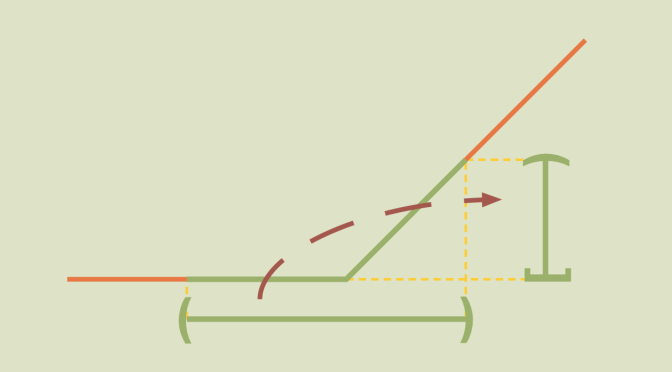# Continuous maps that are not closed or not open

We recall some definitions on open and closed maps. In topology an open map is a function between two topological spaces which maps open sets to open sets. Likewise, a closed map is a function which maps closed sets to closed sets.

For a continuous function $$f: X \mapsto Y$$, the preimage $$f^{-1}(V)$$ of every open set $$V \subseteq Y$$ is an open set which is equivalent to the condition that the preimages of the closed sets (which are the complements of the open subsets) in $$Y$$ are closed in $$X$$. However, a continuous function might not be an open map or a closed map as we prove in following counterexamples.

## A continuous map which is open but not closed

Consider the map:
$\begin{array}{l|lcl} f_1 : & \mathbb{R}^2 & \longrightarrow & \mathbb{R} \\ & (x,y) & \longmapsto & \displaystyle x\end{array}$ $$f_1$$ is continuous as it is a linear mapping between two vector spaces of finite dimensions. Another way to prove $$f_1$$ continuity is to notice the inequality $$\vert x_2- x _1\vert \leq \Vert (x_2,y_2) – (x_1,y_1)\Vert_1$$ where $$\Vert \cdot \Vert_1$$ is the $$\mathbb{R}^2$$-norm: $$\Vert(x,y)\Vert_1 \mapsto \vert x \vert + \vert y \vert$$.

We now prove that $$f_1$$ is open. Suppose that $$U$$ is an open set of $$\mathbb{R}^2$$. We have to prove that $$f_1(U)$$ is open. Consider $$x = f_1((x,y))$$ with $$(x,y) \in U$$. As $$U$$ is open, we can find $$\epsilon > 0$$ such that the open ball $$B_1((x,y),\epsilon)=\{(x^\prime,y^\prime) \in \mathbb{R}^2; \Vert (x^\prime,y^\prime)-(x,y) \Vert_1 < \epsilon \}$$ is included in $$U$$. As $$\vert x^\prime -x \vert \le \epsilon$$ for $$(x^\prime,y^\prime) \in B_1((x,y),\epsilon)$$ the inclusion $$(x-\epsilon,x+\epsilon) \subseteq f_1(U)$$ holds. Hence $$f_1(U)$$ is open being a neighborhood of all its elements. Finally $$f_1$$ is not closed. For the prove we consider the subset $$C = \{(x, \frac{1}{x});x>0\} \subseteq \mathbb{R}^2$$ and the continuous mapping $$\varphi: (x,y) \mapsto xy$$ defined on $$H=\{(x,y) \in \mathbb{R}^2 ; x \ge 0\}$$. $$C$$ is the inverse image $$\varphi^{-1}(\{1\})$$ of the closed subset $$\{1\}$$. Hence $$C$$ is closed in $$H$$. $$C$$ is also closed in $$\mathbb{R}^2$$ as $$H$$ is a closed subset of $$\mathbb{R}^2$$. However, $$f_1(C)$$ is the open ray $$(0,+\infty)$$.

## A continuous map which is closed but not open

Let’s take the real function $$f_2$$ defined as follows:
$f_2(x) = \begin{cases} 0 & \text{if } x < 0 \\ x & \text{if } x \ge 0 \end{cases}$ $$f_2$$ is clearly continuous.

For a subset $$F$$ of the real line, we can write $$F=F_1 \cup F_2$$ where $$F_1=F \cap (-\infty,0)$$ and $$F_2=F \cap [0,+\infty)$$. We have $$f_2(F)=f_2(F_1) \cup f_2(F_2)$$ and $$f_2(F)=F_2$$ if $$F_1=\emptyset$$ whereas $$f_2(F)=\{0\} \cup F_2$$ otherwise. In both cases, $$f_2(F)$$ is closed if $$F$$ is closed. Hence $$f_2$$ is closed.

However, $$f_2$$ is not open as the image of the open set $$(-1,1)$$ is $$[0,1)$$.

## A continuous map which is not open nor closed

For our last conterexample, we take the sine function $$\sin$$. It is well known that $$\sin$$ is continuous.

$$\sin$$ is not open as the image of the open interval $$(0, \pi)$$ is the interval $$(0,1]$$.

$$\sin$$ is also not closed. For the proof which is left to the reader, one can consider the closed subset:
$F=\displaystyle \bigcup_{n \in \mathbb{N}} [2n\pi + \frac{1}{n+1},2n\pi + \pi – \frac{1}{n+1}]$ of the real line and prove that its image by $$\sin$$ is the interval $$(0,1]$$.